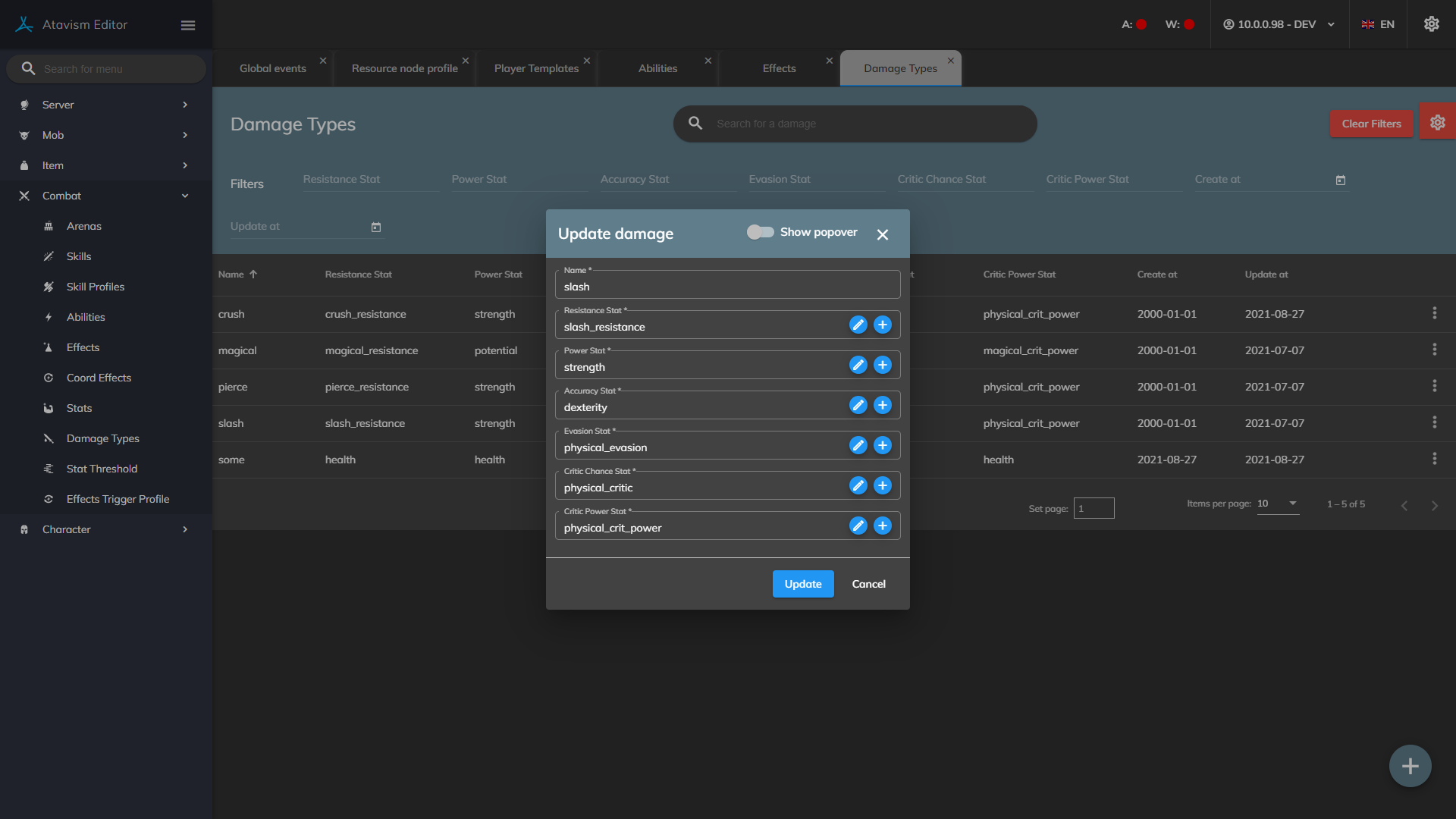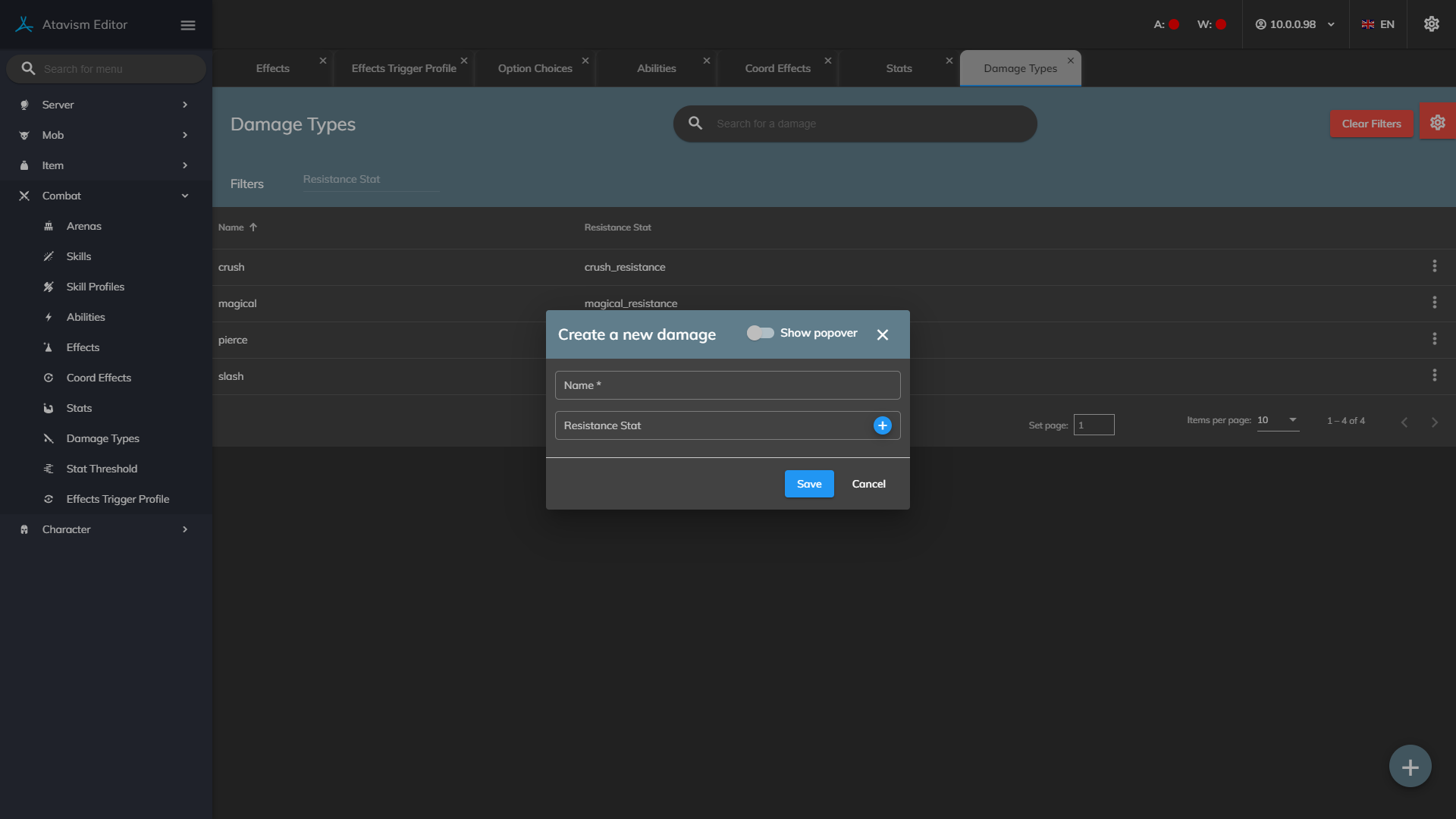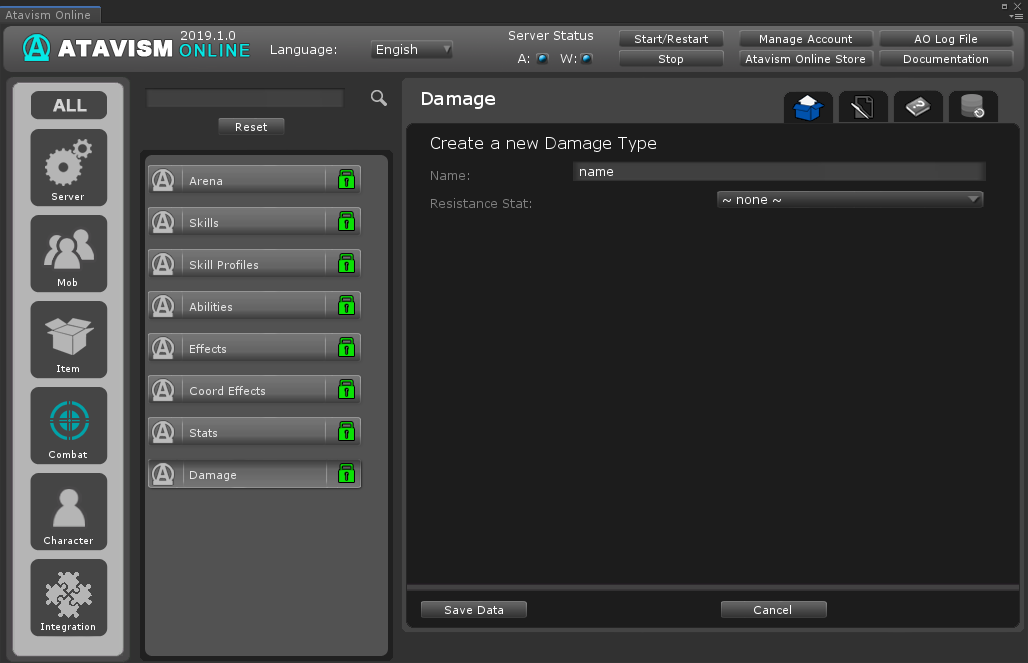Generic filters
Exact matches only
Search in title
Filter by Custom Post Type
Search in project

# Damage Plugin

#### Atavism 10.5.0+Name: The name of the damage type.

Resistance Stat: The resistance stat is used when calculating the damage done for attacks using this damage type.

Power Stat: The power stat is used when calculating the damage done for attacks using this damage type.

Accuracy Stat: The accuracy stat is used when calculating the chance to hit the damage done for attacks using this damage type.

Evasion Stat: The evasion stat is used when calculating the chance to hit the damage done for attacks using this damage type.

Crit Chance Stat: The crit chance stat is used when calculating the chance to hit the damage done for attacks using this damage type.

Crit Power Stat: The resistance stat is used when calculating the damage done for attacks using this damage type.

### Damage is calculated based on this algorithm:

``````DMG_BASE,DMG_MAX - damage from weapon (player) or defined on mob
DMG_AMMO - damage from ammunition
EFFECT_DMG- effect damage
USE_HITROLL - false for DoTs and true for the rest damage effects
POWER_STAT - statistic that is defined in as the power stat for the damage type
DAMAGE_DEALT_MODIFIER - at this point default value is 0
DAMAGE_TAKEN_MODIFIER - at this point default value is 0
HITROLL - random between 0-100
DAMAGE_HITROLL_MODIFIER - game settings global parameter (by default with value 35)
TARGET_ARMOR - target resistance to the attacked damage type
FLAT_ARMOR_DAMAGE_CALCULATIONS - game settings global parameter (by default with value true)
DAMAGE_MOD - damage modifier defined in the effect
SKILL_LEVEL - level of the player's skill assigned to the ability
SKILL_MOD - skill modifier defined in the effect
CRITICAL_CHANCE_CALCULACTED - based on statistic that is defined in as the Critical Chance stat for the damage type using statistic thresholds
CRITICAL_POWER_CALCULATED - based on statistic that is defined in as the Critical Power stat for the damage type using statistic thresholdsDAMAGE_DEALT_MOD - based on statistic that is defined in as the stats module with function Ability Damage Dealt using statistic thresholdsDAMAGE_RECEIVE_MOD - based on statistic that is defined in as the stats module with function Ability Damage Receive using statistic thresholds
BONUS_DMG_EFFECT_VAL - if Bonus Dmg Effect is on the target (from the effect definition) then add Bonus Damage Ammount value
PVP_DAMAGE_REDUCTION_PERCENT - game settings global parameter (by default with value 0.1)
PVP_DAMAGE_REDUCTION_USE - game settings global parameter (by default with value true)

d1 = EFFECT_DMG + random(DMG_BASE,DMG_MAX) + DMG_AMMO

d2= d1 + SKILL_MOD * SKILL_LEVEL

d3 = d2 + d2 * (POWER_STAT / 25)
d4 = d3 + d3 * (DAMAGE_DEALT_MODIFIER/100)

if (USE_HITROLL)
d5 = d4 * ((HITROLL / (100 / DAMAGE_HITROLL_MODIFIER) + (100 - DAMAGE_HITROLL_MODIFIER)) / 100.0)

if FLAT_ARMOR_DAMAGE_CALCULATIONS
d6 = d5 - TARGET_ARMOR
else
if (d5 * 0.99 - TARGET_ARMOR) > 0
d6 = d5 * 0.01 + (d5 * 0.99 - TARGET_ARMOR)
else
d6 = d5 * 0.01

d7 = d6 +d6 * DAMAGE_TAKEN_MODIFIER / 100
if (d7 <= 0)
d7 = 1.0

if (random < (CRITICAL_CHANCE_CALCULACTED / 100f))
d8 = d7 + d7 * CRITICAL_POWER_CALCULATED / 100f

d9 = d8 * DAMAGE_MOD;
d10 = d9 + BONUS_DMG_EFFECT_VAL

RESULT_PARRIED
d11 = d10 * 0.6

if (PVP_DAMAGE_REDUCTION_USE)
d12 = d11 * PVP_DAMAGE_REDUCTION_PERCENT

d13 = Math.round(d12 * DAMAGE_RECEIVE_MOD / 100F * DAMAGE_DEALT_MOD / 100F)

d14 = d13 calculated by Caster TriggerEffect event Type Damage
d15 = d14 calculated by Target TriggerEffect event Type Damage

if RESULT_CRITICAL
d16 = d15 calculated by Caster TriggerEffect event Type Critical
d17 = d16 calculated by Target TriggerEffect event Type Critical

d18 = d17 calculated by Target ShieldEffect
``````

### Hit Chance is calculated based on this algorithm:

``````TARGET_LEVEL - Targer character level
CASTER_LEVEL - Caster character level
HIT_CHANCE_POINT_PER_PERCENTAGE - Global parameter adjustable in the Game Settings Plugin. Defines the number of points per one percent of hit chance
HIT_CHANCE_PERCENTAGE_PER_DIFF_LEVEL - Global parameter is adjustable in the Game Settings Plugin. Defines hit chance reduction by percentage per level difference between defender and attacker
HIT_CHANCE_PERCENTAGE_CAP - Global parameter adjustable int he Game Settings Plugin. Defines maximum chance for miss

STAT_DIFF = ((evasion - accuracy) / HIT_CHANCE_POINT_PER_PERCENTAGE)
if (STAT_DIFF > HIT_CHANCE_PERCENTAGE_CAP)
STAT_DIFF = HIT_CHANCE_PERCENTAGE_CAP
if (STAT_DIFF < 0) STAT_DIFF = 0
int LEVEL_DIFF = (TARGET_LEVEL - CASTER_LEVEL) * HIT_CHANCE_PERCENTAGE_PER_DIFF_LEVEL
if (LEVEL_DIFF < 0) LEVEL_DIFF = 0
HIT_CHANCE= 0.95 - (STAT_DIFF / 100f + LEVEL_DIFF / 100f)``````

### Parry is calculated based on this algorithm:

``````TARGET_LEVEL - Targer character level.
CASTER_LEVEL - Caster character level.
HIT_CHANCE_POINT_PER_PERCENTAGE - Global parameter adjustable in the Game Settings Plugin. Defines the number of points per one percent of hit chance.
HIT_CHANCE_PERCENTAGE_PER_DIFF_LEVEL - Global parameter is adjustable in the Game Settings Plugin. Defines hit chance reduction by percentage per level difference between defender and attacker.
PARRY_PERCENTAGE_CAP - Global parameter adjustable int he Game Settings Plugin. Defines the maximum chance for parry.

STAT_DIFF = ((parry - accuracy) / HIT_CHANCE_POINT_PER_PERCENTAGE)
if (STAT_DIFF > PARRY_PERCENTAGE_CAP)
STAT_DIFF = PARRY_PERCENTAGE_CAP
if (STAT_DIFF < 0) STAT_DIFF = 0
int LEVEL_DIFF = (TARGET_LEVEL - CASTER_LEVEL) * HIT_CHANCE_PERCENTAGE_PER_DIFF_LEVEL
if (LEVEL_DIFF < 0) LEVEL_DIFF = 0
PARRY_CHANCE = 0.05 + (STAT_DIFF / 100f + LEVEL_DIFF / 100f)``````

#### Atavism 10.2.0/10.3.0/10.4.0Name: The name of the damage type.

Resistance Stat: The resistance stat used when calculating the damage done for attacks using this damage type.

Damage is calculated based on this algorithm:

``````dmg-base,dmg-max - damage from weapon (player) or defined on mob
effect_dmg - effect damage
useHitRoll - false for DoTs and true for the rest damage effects
PHYSICAL_POWER_STAT - strength for physical attacks and potential for magical attacks
DAMAGE_DEALT_MODIFIER - at this point default value is 0
DAMAGE_TAKEN_MODIFIER - at this point default value is 0
hitRoll - random between 0-100
DAMAGE_HITROLL_MODIFIER - game settings global parameter (by default with value 35)
targetArmor - target resistance to the attacked damage type
FLAT_ARMOR_DAMAGE_CALCULATIONS - game settings global parameter (by default with value true)
DamageMod - damage modifier defined in the effect
skillMod - skill modifier defined in the effect
criticalChance - character statistic (magical_critic or physical_critic)
bonusDmgEffectVals - if Bonus Dmg Effect is on the target (from the effect definition) then add Bonus Damage Ammount value
PVP_DAMAGE_REDUCTION_PERCENT - game settings global parameter (by default with value 0.1)
PVP_DAMAGE_REDUCTION_USE - game settings global parameter (by default with value true)

d1 = effect_dmg + random(dmg-base,dmg-max)+ ammoDamage

CalcMeleeDamage Start

d2= d1 + skillMod * skillLevel;

d3 = d2 + d2 * (PHYSICAL_POWER_STAT|MAGICAL_POWER_STAT / 25);
d4 = d3 + d3 * (DAMAGE_DEALT_MODIFIER/100);

if (useHitRoll)
d5 = d4 * ((hitRoll / (100 / DAMAGE_HITROLL_MODIFIER) + (100 - DAMAGE_HITROLL_MODIFIER)) / 100.0);

if FLAT_ARMOR_DAMAGE_CALCULATIONS
d6 = d5 - targetArmor;
else
if (d5 * 0.99 - targetArmor) > 0
d6 = d5 * 0.01 + (d5 * 0.99 - targetArmor);
else
d6 = d5 * 0.01

d7 = d6 +d6 * DAMAGE_TAKEN_MODIFIER / 100;
if (d7 <= 0)
d7 = 1.0;

criticalChanceCalculated -  stat PHYSICAL_CRITIC_STAT|MAGICAL_CRITIC_STAT calculated by treshold definition
criticalPowerCalculated  -  stat PHYSICAL_CRITIC_POWER_STAT|MAGICAL_CRITIC_POWER_STAT calculated by treshold definition

if (rand < (criticalChanceCalculated / 100.0))
d8 = d7 + d7 * criticalPowerCalculated / 100f;

CalcMeleeDamage End

d9 = d8 * DamageMod;
d10 = d9 + bonusDmgEffectVals;

RESULT_BLOCKED
d11 = d10 / 2;

RESULT_PARRIED
d11 = d10 * 0.6;

if (PVP_DAMAGE_REDUCTION_USE )
d12 = d11 * PVP_DAMAGE_REDUCTION_PERCENT;

modifyDamage Start

damageDealtMod  - ABILITY_DAMAGE_DEALT_MOD_STAT calculated by treshold definition for statistic
damageReciveMod - ABILITY_DAMAGE_RECEIVE_MOD_STAT  calculated by treshold definition for statistic

d13 = Math.round(d12 * damageReciveMod / 100F * damageDealtMod / 100F)

modifyDamage End

d14 = d13 calculated by Caster TriggerEffect event Type Damage
d15 = d14 calculated by Target TriggerEffect event Type Damage

if RESULT_CRITICAL
d16 = d15 calculated by Caster TriggerEffect event Type Critical
d17 = d16 calculated by Target TriggerEffect event Type Critical

d18 = d17 calculated by Target ShieldEffect
``````

Hit Chance is calculated based on this algorithm:

``````targetLevel - Targer character level.
casterLevel - Caster character level.
HIT_CHANCE_POINT_PER_PERCENTAGE - Global parameter adjustable in the Game Settings Plugin. Defines the number of points per one percent of hit chance.
HIT_CHANCE_PERCENTAGE_PER_DIFF_LEVEL - Global parameter is adjustable in the Game Settings Plugin. Defines hit chance reduction by percentage per level difference between defender and attacker.
HIT_CHANCE_PERCENTAGE_CAP - Global parameter adjustable int he Game Settings Plugin. Defines maximum chance for miss.

statDiff = ((evasion - accuracy) / HIT_CHANCE_POINT_PER_PERCENTAGE);
if (statDiff > HIT_CHANCE_PERCENTAGE_CAP) statDiff = HIT_CHANCE_PERCENTAGE_CAP;
if (statDiff < 0) statDiff = 0;
int levelDiff = (targetLevel - casterLevel) * HIT_CHANCE_PERCENTAGE_PER_DIFF_LEVEL;
if (levelDiff < 0) levelDiff = 0;
hitChance = 0.95 - ( statDiff / 100f + levelDiff / 100f );``````

Parry is calculated based on this algorithm:

``````targetLevel - Targer character level.
casterLevel - Caster character level.
HIT_CHANCE_POINT_PER_PERCENTAGE - Global parameter adjustable in the Game Settings Plugin. Defines the number of points per one percent of hit chance.
HIT_CHANCE_PERCENTAGE_PER_DIFF_LEVEL - Global parameter is adjustable in the Game Settings Plugin. Defines hit chance reduction by percentage per level difference between defender and attacker.
PARRY_PERCENTAGE_CAP - Global parameter adjustable int he Game Settings Plugin. Defines the maximum chance for parry.

statDiff = ((parry - accuracy) / HIT_CHANCE_POINT_PER_PERCENTAGE);
if (statDiff > PARRY_PERCENTAGE_CAP) statDiff = PARRY_PERCENTAGE_CAP;
if (statDiff < 0) statDiff = 0;
int levelDiff = (targetLevel - casterLevel) * HIT_CHANCE_PERCENTAGE_PER_DIFF_LEVEL;
if (levelDiff < 0) levelDiff = 0;
parryChance = 0.05 + ( statDiff / 100f + levelDiff / 100f );``````

#### Atavism 10.1.0-Name: The name of the damage type.

Resistance Stat: The resistance stat used when calculating the damage done for attacks using this damage type.

Damage is calculated based on this algorithm:

``````dmg-base,dmg-max - damage from weapon (player) or defined on mob
effect_dmg - effect damage
useHitRoll - false for DoTs and true for the rest damage effects
PHYSICAL_POWER_STAT - strength for physical attacks and potential for magical attacks
DAMAGE_DEALT_MODIFIER - at this point default value is 0
DAMAGE_TAKEN_MODIFIER - at this point default value is 0
hitRoll - random between 0-100
DAMAGE_HITROLL_MODIFIER - game settings global parameter (by default with value 35)
targetArmor - target resistance to the attacked damage type
FLAT_ARMOR_DAMAGE_CALCULATIONS - game settings global parameter (by default with value true)
DamageMod - damage modifier defined in the effect
skillMod - skill modifier defined in the effect
criticalChance - character statistic (magical_critic or physical_critic)
bonusDmgEffectVals - if Bonus Dmg Effect is on the target (from the effect definition) then add Bonus Damage Ammount value
PVP_DAMAGE_REDUCTION_PERCENT - game settings global parameter (by default with value 0.1)
PVP_DAMAGE_REDUCTION_USE - game settings global parameter (by default with value true)

d1 = effect_dmg + random(dmg-base,dmg-max)+ ammoDamage + skillMod * skillLevel;

d2 = d1 + d1*(PHYSICAL_POWER_STAT / 25);
d3 = d2 + d2 *(DAMAGE_DEALT_MODIFIER/100);

if (useHitRoll)
d3 = d3 *((hitRoll / (100 / DAMAGE_HITROLL_MODIFIER) + (100 - DAMAGE_HITROLL_MODIFIER)) / 100.0);

if FLAT_ARMOR_DAMAGE_CALCULATIONS
d4 = d3*0.01+(d3 * 0.99 - targetArmor);
else
d4 = d3 * ((100-targetArmor) / 100);

d5 = d4 +d4 * DAMAGE_TAKEN_MODIFIER / 100;
if (d5 <= 0)
d5 = 1.0;

criticalChanceCalculated=0;

if (criticalChance <= 440){
criticalChanceCalculated = criticalChance/10;
}
else if(criticalChance > 440 && criticalChance <= 560){
criticalChanceCalculated = (criticalChance - 440) / 20 + 44;
}
else if(criticalChance > 560 && criticalChance <= 660){
criticalChanceCalculated = (criticalChance - 560) / 50 + 44 + 6; //44 from 440/10; 6 from (560 - 440) / 20
}
else if(criticalChance > 660){
criticalChanceCalculated = (criticalChance - 660) / 100 + 44 + 6 + 2; //44 from 440/10; 6 from (560 - 440) / 20; 2 from (660 - 560) / 50
}
if (rand < (criticalChanceCalculated / 100.0))
d6 = d5 * 1.7;

d7 = d6 * DamageMod;
d8 = d7 + bonusDmgEffectVals;

RESULT_BLOCKED
d9 = d8 / 2;

RESULT_PARRIED
d9 = d8 * 0.6;

if (PVP_DAMAGE_REDUCTION_USE )
d10 = d9 * PVP_DAMAGE_REDUCTION_PERCENT;``````

Hit Chance is calculated based on this algorithm:

``````targetLevel - Targer character level.
casterLevel - Caster character level.
HIT_CHANCE_POINT_PER_PERCENTAGE - Global parameter adjustable in the Game Settings Plugin. Defines the number of points per one percent of hit chance.
HIT_CHANCE_PERCENTAGE_PER_DIFF_LEVEL - Global parameter is adjustable in the Game Settings Plugin. Defines hit chance reduction by percentage per level difference between defender and attacker.
HIT_CHANCE_PERCENTAGE_CAP - Global parameter adjustable int he Game Settings Plugin. Defines maximum chance for miss.

statDiff = ((evasion - accuracy) / HIT_CHANCE_POINT_PER_PERCENTAGE);
if (statDiff > HIT_CHANCE_PERCENTAGE_CAP) statDiff = HIT_CHANCE_PERCENTAGE_CAP;
if (statDiff < 0) statDiff = 0;
int levelDiff = (targetLevel - casterLevel) * HIT_CHANCE_PERCENTAGE_PER_DIFF_LEVEL;
if (levelDiff < 0) levelDiff = 0;
hitChance = 0.95 - ( statDiff / 100f + levelDiff / 100f );``````

Parry is calculated based on this algorithm:

``````targetLevel - Targer character level.
casterLevel - Caster character level.
HIT_CHANCE_POINT_PER_PERCENTAGE - Global parameter adjustable in the Game Settings Plugin. Defines the number of points per one percent of hit chance.
HIT_CHANCE_PERCENTAGE_PER_DIFF_LEVEL - Global parameter is adjustable in the Game Settings Plugin. Defines hit chance reduction by percentage per level difference between defender and attacker.
PARRY_PERCENTAGE_CAP - Global parameter adjustable int he Game Settings Plugin. Defines the maximum chance for parry.

statDiff = ((parry - accuracy) / HIT_CHANCE_POINT_PER_PERCENTAGE);
if (statDiff > PARRY_PERCENTAGE_CAP) statDiff = PARRY_PERCENTAGE_CAP;
if (statDiff < 0) statDiff = 0;
int levelDiff = (targetLevel - casterLevel) * HIT_CHANCE_PERCENTAGE_PER_DIFF_LEVEL;
if (levelDiff < 0) levelDiff = 0;
parryChance = 0.05 + ( statDiff / 100f + levelDiff / 100f );``````